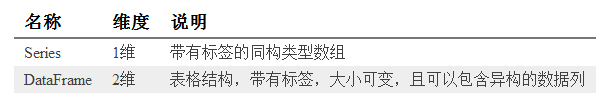# Python 数据处理库 pandas 入门教程基本操作

pandas是一个Python语言的软件包，在我们使用Python语言进行机器学习编程的时候，这是一个非常常用的基础编程库。本文是对Python 数据处理库 pandas 入门教程,非常不错，感兴趣的朋友一起看看吧

pandas是一个Python语言的软件包，在我们使用Python语言进行机器学习编程的时候，这是一个非常常用的基础编程库。本文是对它的一个入门教程。

pandas提供了快速，灵活和富有表现力的数据结构，目的是使“关系”或“标记”数据的工作既简单又直观。它旨在成为在Python中进行实际数据分析的高级构建块。

pandas适合于许多不同类型的数据，包括：

• 具有异构类型列的表格数据，例如SQL表格或Excel数据
• 有序和无序（不一定是固定频率）时间序列数据。
• 具有行列标签的任意矩阵数据（均匀类型或不同类型）
• 任何其他形式的观测/统计数据集。

`sudo pip3 install pandas`

`conda install pandas`

pandas最核心的就是`Series``DataFrame`两个数据结构。DataFrame可以看做是Series的容器，即：一个DataFrame中可以包含若干个Series。

Series

```# data_structure.py
import pandas as pd
import numpy as np
series1 = pd.Series([1, 2, 3, 4])
print("series1:\n{}\n".format(series1))```

```series1:
0 1
1 2
2 3
3 4
dtype: int64```

• 输出的最后一行是Series中数据的类型，这里的数据都是int64类型的。
• 数据在第二列输出，第一列是数据的索引，在pandas中称之为Index。

```# data_structure.py
print("series1.values: {}\n".format(series1.values))
print("series1.index: {}\n".format(series1.index))```

```series1.values: [1 2 3 4]
series1.index: RangeIndex(start=0, stop=4, step=1)```

```# data_structure.py
series2 = pd.Series([1, 2, 3, 4, 5, 6, 7],
index=["C", "D", "E", "F", "G", "A", "B"])
print("series2:\n{}\n".format(series2))
print("E is {}\n".format(series2["E"]))```

```series2:
C 1
D 2
E 3
F 4
G 5
A 6
B 7
dtype: int64
E is 3
DataFrame```

```# data_structure.py
df1 = pd.DataFrame(np.arange(16).reshape(4,4))
print("df1:\n{}\n".format(df1))```

```df1:
0 1 2 3
0 0 1 2 3
1 4 5 6 7
2 8 9 10 11
3 12 13 14 15```

```# data_structure.py
df2 = pd.DataFrame(np.arange(16).reshape(4,4),
columns=["column1", "column2", "column3", "column4"],
index=["a", "b", "c", "d"])
print("df2:\n{}\n".format(df2))```

```df2:
column1 column2 column3 column4
a 0 1 2 3
b 4 5 6 7
c 8 9 10 11
d 12 13 14 15```

```# data_structure.py

df3 = pd.DataFrme({"note" : ["C", "D", "E", "F", "G", "A", "B"],
"weekday": ["Mon", "Tue", "Wed", "Thu", "Fri", "Sat", "Sun"]})
print("df3:\n{}\n".format(df3))```

```df3:
note weekday
0 C Mon
1 D Tue
2 E Wed
3 F Thu
4 G Fri
5 A Sat
6 B Sun```

DataFrame的不同列可以是不同的数据类型

```# data_structure.py
noteSeries = pd.Series(["C", "D", "E", "F", "G", "A", "B"],
index=[1, 2, 3, 4, 5, 6, 7])
weekdaySeries = pd.Series(["Mon", "Tue", "Wed", "Thu", "Fri", "Sat", "Sun"],
index=[1, 2, 3, 4, 5, 6, 7])
df4 = pd.DataFrame([noteSeries, weekdaySeries])
print("df4:\n{}\n".format(df4))```

df4的输出如下：

```df4:
1 2 3 4 5 6 7
0 C D E F G A B
1 Mon Tue Wed Thu Fri Sat Sun```

```# data_structure.py
df3["No."] = pd.Series([1, 2, 3, 4, 5, 6, 7])
print("df3:\n{}\n".format(df3))
del df3["weekday"]
print("df3:\n{}\n".format(df3))```

```df3:
note weekday No.
0 C Mon 1
1 D Tue 2
2 E Wed 3
3 F Thu 4
4 G Fri 5
5 A Sat 6
6 B Sun 7
df3:
note No.
0 C 1
1 D 2
2 E 3
3 F 4
4 G 5
5 A 6
6 B 7```

Index对象与数据访问

pandas的Index对象包含了描述轴的元数据信息。当创建Series或者DataFrame的时候，标签的数组或者序列会被转换成Index。可以通过下面的方式获取到DataFrame的列和行的Index对象：

```# data_structure.py
print("df3.columns\n{}\n".format(df3.columns))
print("df3.index\n{}\n".format(df3.index))```

```df3.columns
Index(['note', 'No.'], dtype='object')
df3.index
RangeIndex(start=0, stop=7, step=1)```

• Index并非集合，因此其中可以包含重复的数据
• Index对象的值是不可以改变，因此可以通过它安全的访问数据

DataFrame提供了下面两个操作符来访问其中的数据：

• loc：通过行和列的索引来访问数据
• iloc：通过行和列的下标来访问数据

```# data_structure.py
print("Note C, D is:\n{}\n".format(df3.loc[[0, 1], "note"]))
print("Note C, D is:\n{}\n".format(df3.iloc[[0, 1], 0]))```

```Note C, D is:
0 C
1 D
Name: note, dtype: object

Note C, D is:
0 C
1 D
Name: note, dtype: object```

`sudo pip3 install xlrd`

```\$ pip3 show xlrd
Name: xlrd
Version: 1.1.0
Summary: Library for developers to extract data from Microsoft Excel (tm) spreadsheet files
Home-page: http://www.python-excel.org/
Author: John Machin
Author-email: sjmachin@lexicon.net
Location: /Library/Frameworks/Python.framework/Versions/3.6/lib/python3.6/site-packages
Requires: ```

```# file_operation.py
import pandas as pd
import numpy as np
print("df1:\n{}\n".format(df1))```

```df1:
C Mon
0 D Tue
1 E Wed
2 F Thu
3 G Fri
4 A Sat
5 B Sun```

```\$ cat test1.csv
C,Mon
D,Tue
E,Wed
F,Thu
G,Fri
A,Sat```

```# file_operation.py
print("df2:\n{}\n".format(df2))```

```\$ cat test2.csv
C|Mon
D|Tue
E|Wed
F|Thu
G|Fri
A|Sat```

```# file_operation.py
print("df3:\n{}\n".format(df3))```

path 文件路径
sep或者delimiter 字段分隔符
index_col 列号或名称用作结果中的行索引
names 结果的列名称列表
skiprows 从起始位置跳过的行数
na_values 代替`NA`的值序列
comment 以行结尾分隔注释的字符
parse_dates 尝试将数据解析为`datetime`。默认为`False`
keep_date_col 如果将列连接到解析日期，保留连接的列。默认为`False`
converters 列的转换器
dayfirst 当解析可以造成歧义的日期时，以内部形式存储。默认为`False`
data_parser 用来解析日期的函数
nrows 从文件开始读取的行数
iterator 返回一个TextParser对象，用于读取部分内容
chunksize 指定读取块的大小
skip_footer 文件末尾需要忽略的行数
verbose 输出各种解析输出的信息
encoding 文件编码
squeeze 如果解析的数据只包含一列，则返回一个`Series`
thousands 千数量的分隔符

```# process_na.py
import pandas as pd
import numpy as np
df = pd.DataFrame([[1.0, np.nan, 3.0, 4.0],
[5.0, np.nan, np.nan, 8.0],
[9.0, np.nan, np.nan, 12.0],
[13.0, np.nan, 15.0, 16.0]])
print("df:\n{}\n".format(df));
print("df:\n{}\n".format(pd.isna(df)));****```

```df:
0 1 2 3
0 1.0 NaN 3.0 4.0
1 5.0 NaN NaN 8.0
2 9.0 NaN NaN 12.0
3 13.0 NaN 15.0 16.0
df:
0 1 2 3
0 False True False False
1 False True True False
2 False True True False
3 False True False False```

```# process_na.py
print("df.dropna():\n{}\n".format(df.dropna()));```

```df.dropna():
Empty DataFrame
Columns: [0, 1, 2, 3]
Index: []```

```# process_na.py
print("df.dropna(axis=1, how='all'):\n{}\n".format(df.dropna(axis=1, how='all')));```

```df.dropna(axis=1, how='all'):
0 2 3
0 1.0 3.0 4.0
1 5.0 NaN 8.0
2 9.0 NaN 12.0
3 13.0 15.0 16.0```

```# process_na.py
print("df.fillna(1):\n{}\n".format(df.fillna(1)));```

```df.fillna(1):
0 1  2  3
0 1.0 1.0 3.0 4.0
1 5.0 1.0 1.0 8.0
2 9.0 1.0 1.0 12.0
3 13.0 1.0 15.0 16.0```

```# process_na.py

df.rename(index={0: 'index1', 1: 'index2', 2: 'index3', 3: 'index4'},
columns={0: 'col1', 1: 'col2', 2: 'col3', 3: 'col4'},
inplace=True);
df.fillna(value={'col2': 2}, inplace=True)
df.fillna(value={'col3': 7}, inplace=True)
print("df:\n{}\n".format(df));```

```df:
col1 col2 col3 col4
index1 1.0 2.0 3.0 4.0
index2 5.0 2.0 7.0 8.0
index3 9.0 2.0 7.0 12.0
index4 13.0 2.0 15.0 16.0```

Series的str字段包含了一系列的函数用来处理字符串。并且，这些函数会自动处理无效值。

```# process_string.py
import pandas as pd
s1 = pd.Series([' 1', '2 ', ' 3 ', '4', '5']);
print("s1.str.rstrip():\n{}\n".format(s1.str.lstrip()))
print("s1.str.strip():\n{}\n".format(s1.str.strip()))
print("s1.str.isdigit():\n{}\n".format(s1.str.isdigit()))```

```s1.str.rstrip():
0  1
1 2
2 3
3  4
4  5
dtype: object
s1.str.strip():
0 1
1 2
2 3
3 4
4 5
dtype: object
s1.str.isdigit():
0 False
1 False
2 False
3  True
4  True
dtype: bool```

```# process_string.py
s2 = pd.Series(['Stairway to Heaven', 'Eruption', 'Freebird',
'Comfortably Numb', 'All Along the Watchtower'])
print("s2.str.lower():\n{}\n".format(s2.str.lower()))
print("s2.str.upper():\n{}\n".format(s2.str.upper()))
print("s2.str.len():\n{}\n".format(s2.str.len()))```

```s2.str.lower():
0   stairway to heaven
1     eruption
2     freebird
3   comfortably numb
4 all along the watchtower
dtype: object

s2.str.upper():
0   STAIRWAY TO HEAVEN
1     ERUPTION
2     FREEBIRD
3   COMFORTABLY NUMB
4 ALL ALONG THE WATCHTOWER
dtype: object

s2.str.len():
0 18
1  8
2  8
3 16
4 24
dtype: int64

```